New Analytical Formulae to Calibrate HPGe Well-type Detectors Efficiency and to Calculate Summing Corrections | OMICS International
Journal of Physical Mathematics

# New Analytical Formulae to Calibrate HPGe Well-type Detectors Efficiency and to Calculate Summing Corrections

Abbas MI1* and Ibrahim OA2

1Physics Department, Faculty of Science, Alexandria University, Alexandria, Egypt

2Physics Department, Faculty of Science, Beirut Arab University, Beirut, Lebanon

*Corresponding Author:
Abbas MI
Physics Department, Faculty of Science
Alexandria University, Alexandria, Egypt
Tel: 2035921675
E-mail: [email protected]

Received Date: March 23, 2015; Accepted Date: April 16, 2015; Published Date: April 26, 2015

Citation: Abbas MI, Ibrahim OA (2015) New Analytical Formulae to Calibrate HPGe Well-type Detectors Efficiency and to Calculate Summing Corrections. J Phys Math 6:134. doi: 10.4172/2090-0902.1000134

Copyright: © 2015 Abbas MI, et al. This is an open-access article distributed under the terms of the Creative Commons Attribution License, which permits unrestricted use, distribution, and reproduction in any medium, provided the original author and source are credited.

Visit for more related articles at Journal of Physical Mathematics

#### Abstract

Direct mathematical method is used in this paper to calculate the photo peak efficiency of a system containing a radioactive source placed in the well of a well type detector. Attenuation of the source and detector’s walls are included. In addition summing corrections are also included. The comparison between published experimental values and present calculated values are given. This work proved quite success in predicting the photo peak efficiency of certain detectors even for a radionuclide emitting rays with different energies.

#### Keywords

Photo peak efficiency; Detectors; Photons; Azimuth angle

#### Introduction

The direct mathematical method is an analytical method created by Younis S. Selim and Mahmoud I. Abbas to calculate the total or photo peak efficiency of radioactive detectors [1-4].Then it was used by several authors. The comparison between the analytical values and experimental ones shows that the error is very small. Besides, the direct mathematical method is built on simple physical concept and mathematical integrals and it does not need long computer programs like other analytical methods. This analytical method is used to calculate the photo peak efficiency of a radioactive source placed in the well of a well type HPGe detector.

In this paper, the direct mathematical method is explained, then a detailed mathematical perspective is described and a comparison between analytical, experimental and those obtained by Monte Carlo method is given. In addition, new method to correct for summing corrections is discussed.

¨Description of our system

Our system is made of a well type semiconductor detector made by Canberra. Its active material (Ge) is mounted in aluminum end cap of thickness 0.5 mm and having an external shielding of lead. The source should be put in the detector well; it can be a “point source” with very small size or a volumetric source that can fit in the well.

The radioactive source contains radioactive nuclide emitting gamma rays. Each nuclide can emit gamma rays with specific energy; the rays emitted can be attenuated by the source material and container walls as well as the detector’s walls. Other rays will be absorbed by the detector material. The rays absorbed with in the detector active medium will deposit their energy in it. A ray can deposit all its energy or part of it depending on the way of interaction. The energy deposited will be turned into an electric signal. This electric signal is sent to an amplifier, then to a multichannel analyzer that will record the pulse. Finally a computer will display all the signals depending on their energy Figure 1.

The total efficiency of the system is defined to be the total number of rays detected divided by the total number of rays emitted while the photo peak efficiency is defined to be the number of rays detected within certain energy divided by the number of rays emitted at this energy. In this work we will focus on photo peak efficiency .

Direct mathematical method

This method uses simple mathematical idea to calculate total efficiency as well as photo peak efficiency. It starts from this perspective, when a photon enters the detector active medium, the probability for it to not interact with the detector is given by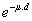where μ is the linear attenuation coefficient expressed in cm-1 and d is the distance covered in the detector active medium. So the probability for a photon striking a detector to interact with its material is done by: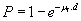(1)

Where, is the total attenuation coefficient and it depends on the detector material type and photon’s energy. Also the photon might be absorbed by the walls of the detector or by the source material itself. The probability to be absorbed by certain non-detecting material is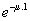. The “attenuation factor” caused by several walls or barriers can be done by [6-10]: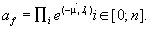(2)

Where,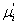is the attenuation coefficient of the ith absorber and li is the distance covered in that absorber. So in general, the probability for a single photon, to cross n barriers then enter the detector material, and interact with it depositing certain energy will be: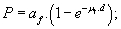(3)

While the probability of the same photon to be interacted with the detector depositing all its energy will be: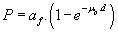(4)

Where, μp is called the photo peak attenuation coefficient. The interactions contributing to determine μp are: photoelectric effect and pair production. While Compton scattering does not contribute unless followed by photoelectric effect. Measuring efficiency for certain system requires two main things, the first one is full awareness of the geometry of the system to calculate the distance a photon emitted at certain polar and azimuth angle will cover in detecting and attenuating materials. Besides it requires understanding the different kinds of interactions between the photon and these materials. In addition, detector dead time and summing out effects must be known also.

So for a point source the efficiency in general will be the sum ‘integration” of all rays detected at any polar or azimuth angle divided by the total number of rays emitted at certain energy or the integrationsum of the probability function (equation.3) over the entire solid angle divided by the total solid angle: 4π. The general rule is: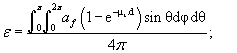(5)

To calculate the photo peak efficiency of the same point source, we replace μt by μp.

#### Mathematical Perspective

Axial point source

To derive an equation for this efficiency, we will start by studying the different path lengths allowed for a photon starting from a point source. Then we will write a mathematical equation covering the entire solid angle, which is a developed form of equation 5 to deal with different functions of the distance covered in the detector “d”.

Allowed path lengths in detecting and attenuating materials:

For a photon created by an axial point source, five path lengths are allowed for a photon in the detecting material. All the path lengths functions are illustrated in Figures 2 and 3.

Figure 2: Drawing not to scale showing to the left a projection on a vertical plane passing through axis not to scale and to the right a three dimensional drawing of the detector and the four path lengths allowed for a photon emitted from a source located at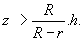Figure 3: Three dimensional drawing not to scale of our detector showing the four path length allowed for a photon emitted from a point source located at height ’z“less than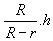1. If the photon enters the detector from the well’s bottom and leaves from the bottom the path length “d1” followed will be:

d1 =h/cos (θ) (6)

2. If the photon enters from the well’s bottom and leaves from the outer side, the path length function will be: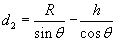(7)

3. If the photon enters the detector material from the well’s side and leaves from the bottom: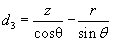(8)

4. If the photon enters the detector from the well’s side and leaves from the outer side. The path length “d3” followed will be: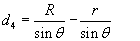(9)

If the photon enters from the well’s side and leaves from the face, the path length function will be: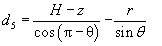(10)

While the distance traveled by the photon in the non-detecting materials usually source container or detector shielding is simply,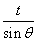if the photon crosses the wall of source or shielding; and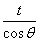if the photon is crossing the bottom of the container or the well’s bottom; t is the thickness of the absorber.

Photo peak efficiency: Since we are dealing with an axial point source, the azimuth angle does not affect the path length of a photon, the later depends only on the polar angle. We will start our study by defining four polar angles that are important to our study.

Which are: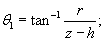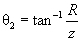;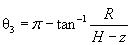;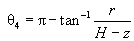. These polar angles are shown in Figure 2.

We have to discuss two cases:

A.If the point is located at a height more than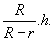(Figure 2):

For 0<θ<θ1; the photon has to follow path length: d1.

For θ1<θ<θ2; the photon has to follow path length: d3

For θ2<θ<θ3; the photon has to follow path length: d4.

For θ3<θ<θ4; the photon has to follow path length: d5.

For θ4<θ <π; the photon will never strike the detector.

So the photo peak efficiency is given by: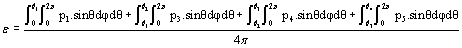(11)

Where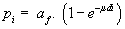B. If the point source located at a height less than.(Figure 3):

For 0<θ<θ2; the photon has to follow the path length: d1.

For θ2<θ<θ1; the photon has to follow the path length: d2.

For θ1<θ<θ3; the photon has to follow the path length: d4.

For θ3<θ<θ4; the photon has to follow the path length: d5.

For θ3<θ<π; the photon will never strike the detector.

In this case the photo peak efficiency will be: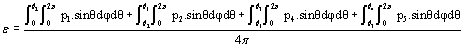(12)

Non Axial point Source

For a point source not located on the axis of detector the path length functions allowed depend on two things only where the photon enters the detecting material and where it leaves. These things in turn depend on the location of the source (its height “z” and the axial distance “ρ”) as well as the polar and azimuth angle (Figures 4-6).

Figure 4: Three dimensional drawing not to scale of our detector showing the four path length allowed for a photon emitted from a point source located at height “z’less than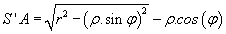as it is obvious by simple geometry of triangle AHO.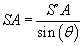Figure 5: Clarifies the path length d4’. The solid segment AB “to the left” represents the distance covered in HPGe Material. By projecting on a horizontal plane “to the right”we obtain A’B’ the projection of AB.

Figure 6: Shows a projection on a horizontal plane containing the well bottom to discuss the ± in the equation of d2’ according to this figure. This “±” turns – for φ<φc and + for φ>φc.

Allowed path Lengths: In general for a photon generated at a point source S (ρ; z), the path length it can follow can be:

• If it enters from the well’s bottom and leaves from the bottom. The path length it can follow is d1 (as identified before in equation 6).

• If it enters from the well’s bottom and leaves from the side: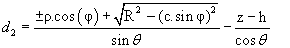(13)

If it enters from the side and leaves from the bottom:(14)

4. If it enters from the side and leaves from the side: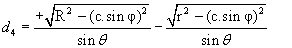(15)

A’B’ is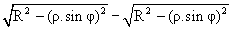5. If it enters from the side and leaves from the upper face: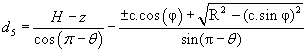(16)

Photo peak Efficiency: Studying the photo peak efficiency of a non-axial point source is more complicated than the axial source case, because we will study the path length functions allowed according to polar and azimuth angle both range from 0 to π. We define four new polar angles illustrated in Figure 7:

Figure 7: Shows a three dimensional drawings of our system with the four angles θ1; θ2; θ3 and θ4 to the left and a projection on a horizontal plane c ontaining the well’s bottom. Equation of φc’can be applying the cosine law in the triangle S’AO:

Figure 8: Shows to the left a 3 Dimensionalillustration the polar angles: θ5; θ6; θ7 and θ8.Projection of these polar angles θ5; θ6 θ7 and θ8 on the upper surface of the well and detector is shown to the right. Also φm and φm’ are shown for two random angles. The simple geometry of triangles S’OA and S’OB allow us to derive the equations of φm and φm’.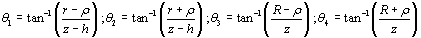In the lower part of the detector (located below the point source)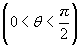A projection is shown in Figure 7 on a horizontal plane containing the well’s bottom to understand the different places the photon can enter from.

According to this projection:

For 0<θ1, the photon has to enter from the well’s bottom.

For θ1<θ<θ2, the photon can enter either from the well’s bottom or well’s side depending on the azimuth angle: φ.

If φ <φ’c ; “illustrated in figure 7” the photon enters from the well’s bottom.

If φ >φ’c; the photon enters from the well’s wall.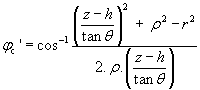(17)

For θ>θ2; the photon must enter from the wall.

A similar projection on a horizontal plane containing the detector’s bottom can show where the photon can show the detector:

For 0<θ3, the photon has to leave from the bottom

For θ3<θ<θ4.the photon can leave either from the bottom or from the wall depending on the azimuth angle: φ.

If φ <φc; “indicated in figure” the photon leaves from the wall

If φ >φc; the photon leaves from the wall.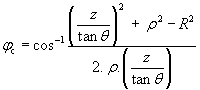(18)

For θ> θ4, the photon must leave from the wall.

Note that: the equation of φc can be obtained similar way as φ’c by just replacing “r” by “R” and “z-h” by “z” shown in Table 1.

0<θ3 θ3<θ<θ4
φ <φ’c
θ3<θ<θ4
φ >φ’c
θ4< θ<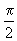0<θ1 d1 d2 d1 d2
θ1<θ<θ2
φ <φc
d3 d4 d3 d4
θ1<θ<θ2
φ >φc
d1 d2 d1 d2
θ2<θ<d3 d4 d3 d4

Table 1: shows the path length followed by the photon depending on its polar and azimuth angle for θ <.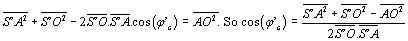In the upper part of the detector (located above the point source)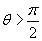:

To study the path length function followed by a photon striking the upper part of the detector we will start by defining five new angles: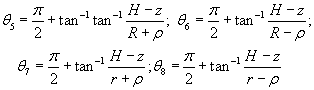For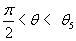; the photon must enter from the well’s wall and leave from the detector’s wall so it must follow path length d4’.

For θ5<θ<θ6; the photon must enter from the wall but it can leave either from upper side or wall depending on the azimuth angle φ. It leaves from upper face for φ<φm and from the wall for φ>φm.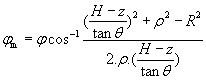(19)

For θ6<θ< θ7; the photon must enter from the well’s wall and leave from the detector’s upper surface following path length d5’.

For θ7<θ<θ8; the photon can enter the detector from the wall and leave from upper face following path length d5’ and not strike the detector for φ >φm’ shown in Table 2.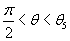θ5<θ< θ6 θ6<θ<θ7 θ7<θ<θ8
φ<φm d4 d4 d5 d5
Φm’<φ d4 d5 d5 d5
φ<φm d4 d5 d5 d5
φ>φm d4 d5 d5 0

Table 2: Shows the different path length functions allowed for a photon crossing the upper part of the detector ( θ >).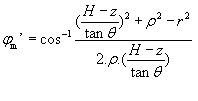(20)

For θ>θ8; the photons leave without touching the detecting material. So the point source efficiency will be: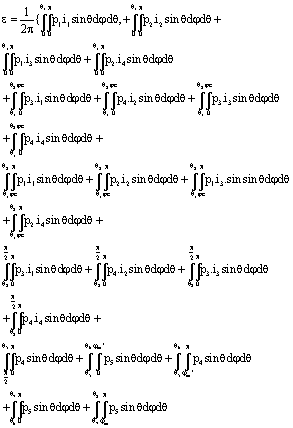(21)

Where, i1is a function that turns to 1 when θ<θ3 and turns to zero if else.i2 is a function that turns to 1 when θ3<θ<θ4 and simultaneously φ<φc’; but turns to zero if else., i3 is a function that turns to 1 when θ3<θ<θ4 and φ>φc’; but turns to zero if else., i4 is a function that turns to 1 if θ>θ4 and turns to zero if else.

Efficiency of a cylindrical source

The efficiency of a coaxial disc source of radius S (S<r) placed inside the well can be done by integrating over dρ and ρ ranges from 0 to S. the integration over dφ is useless because of the symmetry: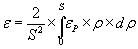(22)

Where εp is the efficiency of a point source as identified before in equation 21.In case of cylindrical sources of radius S and height H, we have to integrate over dρ and dh: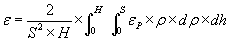(23)

This equation in its developed form was used to calculate the theoretical values represented in Tables 3 and 4.

E (keV) Expt.  Present work M.C. 
46.5 67.68 61.2 72.77
59.5 72.3 73.3 77.1
88 76.75 75.3 80.87
122 73 74.7 77.8
279 40.5 41.4 41.99
392 28.26 28.5 30.09
514 22.1 21.6 22.79
661 17.76 16.9 18.56
898 7.64 7.7 8.34
1173 5.6 5.6 6.25
1332 4.77 4.63 5.46
1863 3.45 2.9 3.82

Table 3: Comparison between different values calculated using our program with experimental ones for a source constituting of a plastic vial of diameter 1.5 cm containing 3 cm3 of water in which the following radioactive nuclides are dissolved.

E (keV) Expt. Present work M.C. 
59.5 59.9 58.3 64.4
88 60.5 64.3 66.06
122 56.35 63.4 64.3
279 31.08 31.1 33.98
392 21.85 23.6 24.25
514 16.86 18.06 18.57
661 13.78 14.2 14.84
834 11.02 11.2 12.21
898 6.43 6.4 7.5
1173 4.72 5.1 5.57
1333 4.1 4.41 4.62
1863 3.03 2.63 3.32

Table 4: Comparison between different values calculated using our program, Monte Carlo method and experimental ones for a source with 8 cm3 volume of water that contains same radionuclides.

#### Summing out Correction

Since we are dealing with photo peak efficiency; it is crucial to be aware of the signals resulting from the simultaneous detection of two rays. The result will be a pulse recorded within a channel of height corresponding to the sum of energies of both rays. This is frequent when a radioactive isotope emitting two or more gamma lines in its decay. There is a possibility that one ray will interact with the detector material depositing all of its energy in it, so it is expected to be recorded in the full-energy peak (photo peak), but the other might deposit part of its energy or all of it. Thus the total energy deposited will be higher than the one corresponding to the full energy peak of the first and the first ray will never be recorded in the full-energy peak.

In our program we added the effect of the summing correction. For example Cobalt-60 emits simultaneously two gamma lines with energies 1173 and 1332keV. According to equation5, the probability of a 1173keVgamma line to be recorded in the full-energy peak should be af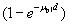, where μp1 is the photo peak attenuation coefficient of the photons having 1173keV energy. This probability will decrease because of the 1332keV gamma line is depositing its energy. The probability of 1332-ray to escape the detector will be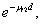where μt2 here is the total attenuation coefficient of the photons having 1332 keV energy. So equation 5 turns to: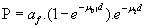(24)

#### Results and Discussion

Equations 11, 12, 23 and 24were used to get the efficiency of point sources and cylindrical sources. Comparison between the calculated values in our work, those calculated using Monte Carlo method and experimental values is tabulated in the following table.

210Pb (46 keV), 241Am (59 keV), 109Cd (88 keV), 57Co (122 keV),203 Hg (279 keV), 113Sn (391 keV), 85Sr (514 keV), 137Cs (661 keV), 54Mn (834 keV), 88Y (898 and 1836 keV) and 60Co (1173 and 1332 keV).

#### Conclusion

The present work offers straightforward mathematical expressions to calibrate HPGe well type detectors over a large energy range without the need for standard sources, norlong computer programs as for other simulation methods. In addition, the summing corrections are also presented in a simple mathematical expression. Tables 3 and 4 shows the differences between analytical and experimental values are very small. Direct mathematical method proves quite success even when some radionuclides placed in the well of the detector emit several gamma lines.

#### References

Select your language of interest to view the total content in your interested language

### Article Usage

• Total views: 13207
• [From(publication date):
April-2015 - Jan 18, 2020]
• Breakdown by view type
• HTML page views : 9390Can't read the image? click here to refresh